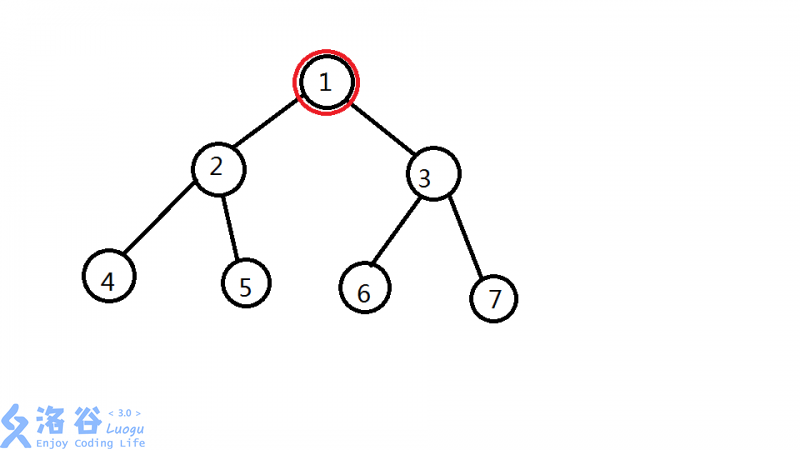# P5002 专心OI - 找祖先

• 544通过
• 2.1K提交
• 题目提供者 Imakf
• 评测方式 云端评测
• 标签 搜索 最近公共祖先,LCA 枚举,暴力
• 难度 提高+/省选-
• 时空限制 1000ms / 128MB
• 提示：收藏到任务计划后，可在首页查看。

# 最后一个点15pts

Imakf是一个小蒟蒻，他最近刚学了LCA，他在手机APP里看到一个游戏也叫做LCA就下载了下来。

## 题目描述

这个游戏会给出你一棵树，这棵树有$N$个节点，根结点是$R$，系统会选中$M$个点$P_1,P_2...P_M$，要Imakf回答有多少组点对$(u_i,v_i)$的最近公共祖先是$P_i$。Imakf是个小蒟蒻，他就算学了LCA也做不出，于是只好求助您了。

Imakf毕竟学过一点OI,所以他允许您把答案模 $(10^9+7)$

## 输入输出格式

输入格式：

第一行 $N , R , M$

此后$N-1$行 每行两个数$a,b$ 表示$a,b$之间有一条边

此后$1$行 $M$个数 表示$P_i$

输出格式：

$M$行，每行一个数，第$i$行的数表示有多少组点对$(u_i,v_i)$的最近公共祖先是$P_i$

## 输入输出样例

输入样例#1： 复制
7 1 3
1 2
1 3
2 4
2 5
3 6
3 7
1 2 4
输出样例#1： 复制
31
7
1

## 说明

样例1

对于询问1 $~(1,1) (1,2) (1,3) (1,4) (1,5) (1,6) (1,7) (2,1) (2,3) (2,6) (2,7) (3,1) (3,2) (3,4) (3,5) (4,1) (4,3)$

$(4,6) (4,7) (5,1) (5,3) (5,6) (5,7) (6,1) (6,2) (6,4) (6,5) (7,1) (7,2) (7,4) (7,5)$共31组

询问2 $(2,2) (2,4) (2,5) (4,2) (4,5) (5,2) (5,4)$共7组

对于询问3 $(4,4)$共1组

$N\leq10000,M\leq50000$提示
标程仅供做题后或实在无思路时参考。
请自觉、自律地使用该功能并请对自己的学习负责。
如果发现恶意抄袭标程，将按照I类违反进行处理。